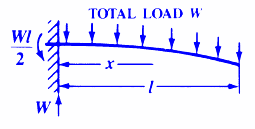Bending, Deflection and Stress Equations Calculator for

Beam Deflection and Stress Formula and Calculators

Structural Beam Deflection, Stress, Bending Equations and calculator for a Cantilevered Beam with Uniform Load.Stress at specific pointStress at the support (must be constant cross section)Deflection at specified pointDeflection at the unsupported end, With Total load "W"or

Deflection at the unsupported end, With Line Pressure Load "p"Where:

 E = Modulus of Elasticity psi (N/mm2) I = Moment of Inertia in4 (mm4) W = Total Load on Beam (Not Line Load) lbs (N) p = With Line Pressure Load psi-in (N/mm) s = Stress at the cross-section being evaluated Lbs/in2 (N/mm2) y = Deflection inches (mm) x = Some distance as indicated inches (mm) Z = section modulus of the cross-section of the beam = I/z in3 (mm3) z = distance from neutral axis to extreme fiber (edge) inches (mm)= Length as indicated inches (mm)
• Please note letter "" (lower case "L") is different than "I" (Moment of Inertia).
• Deflections apply only to constant cross sections along entire length.

References:

• Any Machinery's Handbook published since 1931 or,
• Machinery's Handbook, 21st Edition, Page 407 or,
• Machinery's Handbook, 23st Edition, Page 263 or,
• Machinery's Handbook, 27st Edition, Page 264
•Membership Register | LoginHomeEngineering Book StoreEngineering ForumExcel App. DownloadsOnline Books & ManualsEngineering NewsEngineering VideosEngineering CalculatorsEngineering ToolboxGD&T Training Geometric Dimensioning TolerancingDFM DFA TrainingTraining Online EngineeringAdvertising CenterCopyright Notice